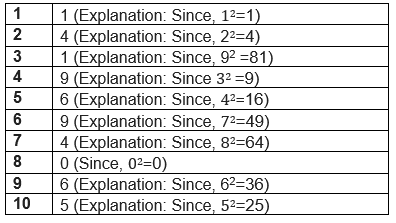# NCERT Solutions for Class 8 Maths Chapter 6 Square and Square roots CBSE Exercise 6.1

In this page we have NCERT Solutions for Class 8 Maths Chapter 6 Exercise 6.1 .This exercise has questions about Pattern of numbers , properties of the square number, how to find the unit digit of the square. Hope you like them and do not forget to like , social share and comment at the end of the page.

## NCERT Solutions for Class 8 Maths Chapter 6 Exercise 6.1

Question 1
What will be the unit digit of the squares of the following numbers?
(i) 81
(ii) 272
(iii) 799
(iv) 3853
(v) 1234
(vi) 26387
(vii) 52698
(viii) 99880
(ix) 12796
(x) 55555
The unit digit square decide the number for the square of any numberQuestion 2
The following numbers are obviously not perfect squares. Give reason.
1. 1057
2. 23453
3. 7928
4. 222222
5. 64000
6. 89722
7. 222000
8. 505050
The square of any number will have 0,1,4,5,6 or 9 at its unit place
So (i), (ii), (iii), (iv), (vi) don’t have any of the 0, 1, 4, 5, 6, or 9 at unit’s place, so they are not be perfect squares.
The square of Zeros will be even always
So (v), (vii) and (viii) don’t have even number of zeroes at the end so they are not perfect squares.

Question 3
The squares of which of the following would be odd numbers?
1. 431
2. 2826
3. 7779
4. 82004
1. 431 square will end in 1,So odd number
2. 2826 square will end in 6 ,so even number
3. 779 square will end in 1,So odd number
4. 82004 square will end in 6 ,so even number

Question 4
Observe the following pattern and find the missing digits.
$11^2= 121$
$101^2= 10201$
$1001^2= 1002001$
$100001^2$= 1.........2.......1
$10000001^2$= ...............
Solution:
$100001^2= 10000200001$
$10000001^2= 100000020000001$
Reasoning Start with 1 followed as many zeroes as there are between the first and the last one, followed by two again followed by as many zeroes and end with 1.

Question 5
Observe the following pattern and supply the missing numbers.
$11^2= 121$
$101^2= 10201$
$10101^2= 102030201$
$1010101^2$= ..................
..............2=$10203040504030201$
$1010101^2= 1020304030201$
$101010101^2=10203040504030201$
Reasoning Start with 1 followed by a zero and go up to as many number as there are number of 1s given, follow the same pattern in reverse order.

Question 6
Using the given pattern, find the missing numbers.
$1^2+ 2^2+ 2^2= 3^2$
$2^2+ 3^2+ 6^2= 7^2$
$3^2+ 4^2+ 12^2= 13^2$
42+ 52+ _2= 212
52+ _2+ 302= 312
62+ 72+ _2= _2
We can see following pattern the above series
Relation among first, second and third number - Third number is the product of first and second number
Relation between third and fourth number - Fourth number is 1 more than the third number
So
$4^2+ 5^2+ 20^2= 21^2$
$5^2+ 6^2+ 30^2= 31^2$
$6^2+ 7^2+ 42^2= 43^2$

Question 7
(i) $1 + 3 + 5 + 7 + 9$
(ii) $1 + 3 + 5 + 7 + 9 + I1 + 13 + 15 + 17 +19$
(iii) $1 + 3 + 5 + 7 + 9 + 11 + 13 + 15 + 17 + 19 + 21 + 23$
Explanation:
$1 + 3 = 2^2= 4$
$1 + 3 + 5 = 3^2= 9$
$1 + 3 + 5 + 7 = 4^2=16$
$1 + 3 + 5 + 7 + 9 = 5^2= 25$
So Sum of n odd numbers starting from 1 = $n^2$
From the above derivation we can answer the above questions
1. Since, there are 5 consecutive odd numbers, Thus, their sum = $5^2= 25$
2. Since, there are 10 consecutive odd numbers, Thus, their sum = $10^2= 100$
3. Since, there are 12 consecutive odd numbers, Thus, their sum = $12^2= 144$

Question 8
(i) Express 49 as the sum of 7 odd numbers.
(ii) Express 121 as the sum of 11 odd numbers.
Explanation:
$1 + 3 = 2^2= 4$
$1 + 3 + 5 = 3^2= 9$
$1 + 3 + 5 + 7 = 4^2=16$
$1 + 3 + 5 + 7 + 9 = 5^2= 25$
So Sum of n odd numbers starting from 1 = $n^2$
(1) Since, $49 = 7^2$
So, $7^2$ can be expressed as follows:
$1 + 3 + 5 + 7 + 9 + 11 + 13$
(2) Since, $121 = 11^2$
Therefore, $121 = 1 + 3 + 5 + 7 + 9 + 11 + 13 + 15 + 17 + 19 + 21$

Question 9
How many numbers lie between squares of the following numbers?
(i) 12 and 13
(ii) 25 and 26
(iii) 99 and 100
i. $12^2= 144$
$13^2= 169$
Now, 169 - 144 = 25
So, there are 25 - 1 = 24 numbers lying between $12^2$ and $13^2$
ii. We know that, $25^2= 625$
And, $26^2= 676$
Now, 676 - 625 = 51
So, there are 51 - 1 = 50 numbers lying between $25^2$ and $26^2$
iii. We know that, $99^2= 9801$
And, $100^2= 10000$
Now, $10000 - 9801 = 199$
So, there are 199 - 1 = 198 numbers lying between $99^2$ and $100^2$

## Summary

1. NCERT Solutions for Class 8 Maths Chapter 6 Exercise 6.1 has been prepared by Expert with utmost care. If you find any mistake.Please do provide feedback on mail. You can download the solutions as PDF in the below Link also
2. This chapter 6 has total 4 Exercise 6.1 ,6.2,6.3 and 6.4. This is the First exercise in the chapter.You can explore previous exercise of this chapter by clicking the link below